What will be the equivalent resistance between the two points A and D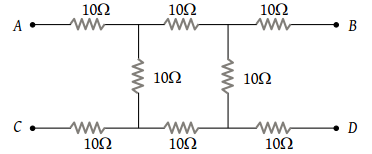(1) 10 Ω

(2) 20 Ω

(3) 30 Ω

(4) 40 Ω

Concept Questions :-

Combination of resistors
High Yielding Test Series + Question Bank - NEET 2020

Difficulty Level:

What is the equivalent resistance between A and B in the figure below if R = 3 Ω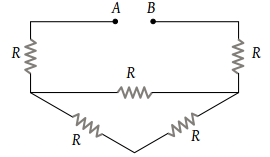(1) 9 Ω

(2) 12 Ω

(3) 15 Ω

(4) None of these

Concept Questions :-

Combination of resistors
High Yielding Test Series + Question Bank - NEET 2020

Difficulty Level:

What is the equivalent resistance between A and B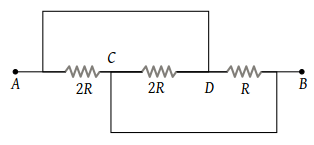(1) $\frac{2}{3}R$

(2) $\frac{3}{2}R$

(3) $\frac{R}{2}$

(4) 2 R

Concept Questions :-

Combination of resistors
High Yielding Test Series + Question Bank - NEET 2020

Difficulty Level:

The current in the following circuit is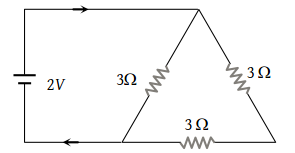(1) $\frac{1}{8}A$

(2) $\frac{2}{9}A$

(3) $\frac{2}{3}A$

(4) 1A

Concept Questions :-

Combination of resistors
High Yielding Test Series + Question Bank - NEET 2020

Difficulty Level:

What is the equivalent resistance of the circuit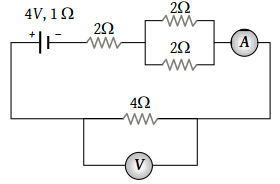(1) 6 Ω

(2) 7 Ω

(3) 8 Ω

(4) 9 Ω

Concept Questions :-

Combination of resistors
High Yielding Test Series + Question Bank - NEET 2020

Difficulty Level:

The equivalent resistance of the circuit shown in the figure is :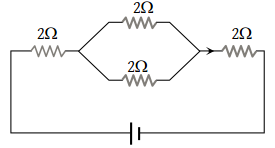(1) 8 Ω

(2) 6 Ω

(3) 5 Ω

(4) 4 Ω

Concept Questions :-

Combination of resistors
High Yielding Test Series + Question Bank - NEET 2020

Difficulty Level:

In the given figure, the equivalent resistance between the points A and B is :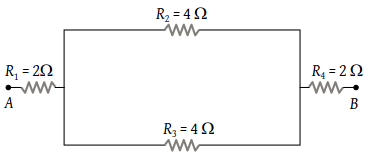(1) 8 Ω

(2) 6 Ω

(3) 4 Ω

(4) 2 Ω

Concept Questions :-

Combination of resistors
High Yielding Test Series + Question Bank - NEET 2020

Difficulty Level:

An infinite ladder network is arranged with resistances R and 2 R as shown. The effective resistance between terminals A and B is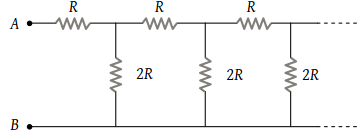(1) ∞

(2) R

(3) 2 R

(4) 3 R

Concept Questions :-

Combination of resistors
High Yielding Test Series + Question Bank - NEET 2020

Difficulty Level:

A battery of emf 10 V and internal resistance 3 Ω is connected to a resistor as shown in the figure. If the current in the circuit is 0.5 A. then the resistance of the resistor will be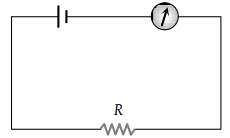(1) 19 Ω

(2) 17 Ω

(3) 10 Ω

(4) 12 Ω

Concept Questions :-

Kirchoff's voltage law
High Yielding Test Series + Question Bank - NEET 2020

Difficulty Level:

The potential drop across the 3Ω resistor is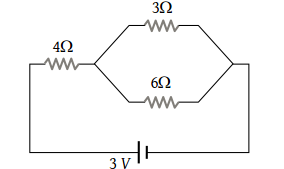(1) 1 V

(2) 1.5 V

(3) 2 V

(4) 3 V

Concept Questions :-

Combination of resistors